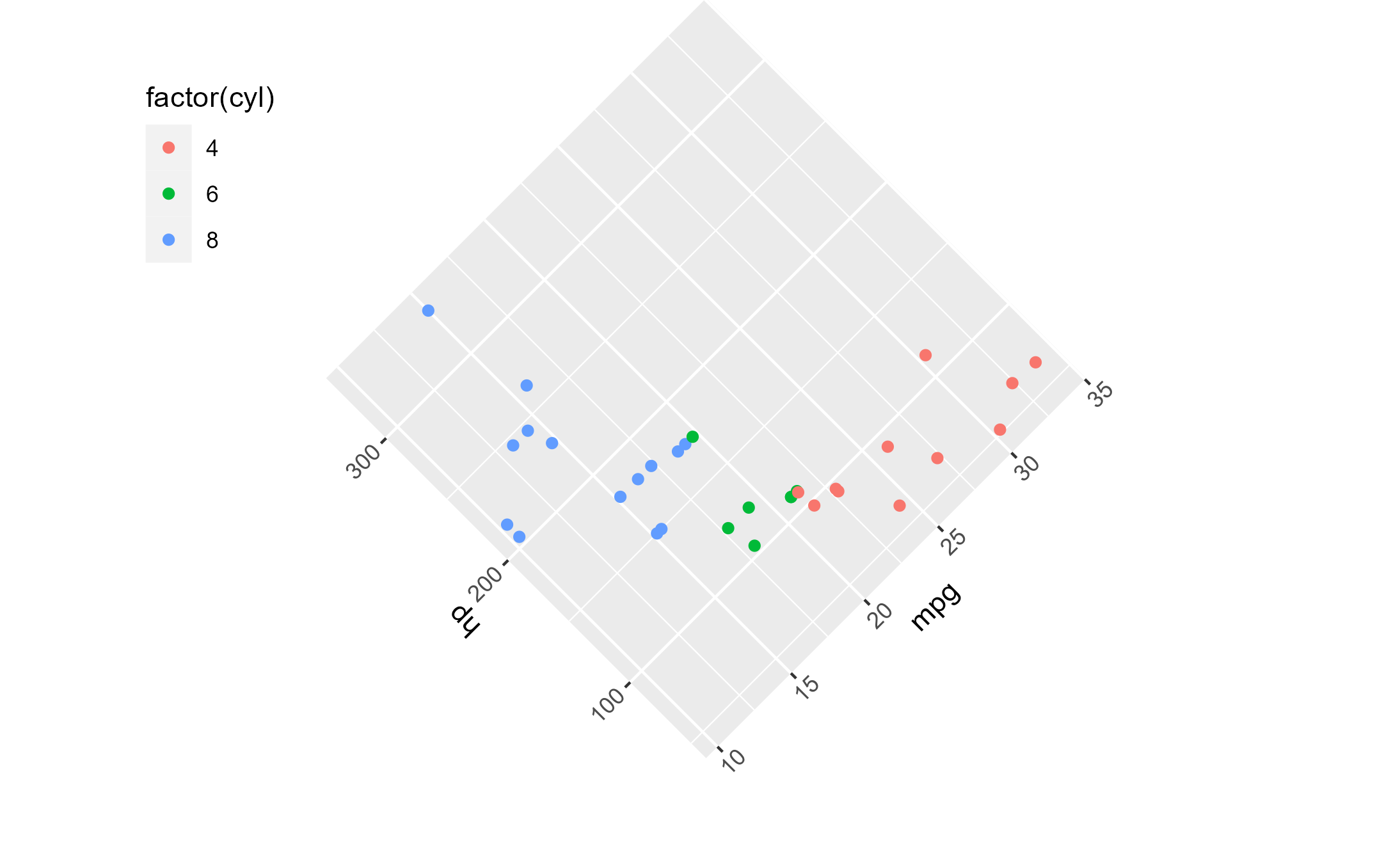with_ggtrace() provides a functional interface to ggtrace(). It takes a ggplot object and parameters passed to ggtrace() and returns the immediate tracedump and/or graphical output without side effects.

## Usage

with_ggtrace(x, method, ..., out = c("tracedump", "gtable", "both"))

## Arguments

x

A ggplot object whose evaluation triggers the trace as specified by the ...

method

A function or a ggproto method. The ggproto method may be specified using any of the following forms:

• ggproto$method • namespace::ggproto$method

• namespace:::ggproto$method ... Arguments passed on to ggtrace trace_steps A sorted numeric vector of positions in the method's body to trace. Negative indices reference steps from the last, where -1 references the last step in the body. trace_exprs A list of expressions to evaluate at each position specified in trace_steps. If a single expression is provided, it is recycled to match the length of trace_steps. To simply run a step and return its output, you can use the ~step keyword. If the step is an assign expression, the value of the assigned variable is returned. If trace_exprs is not provided, ggtrace() is called with ~step by default. once Whether to untrace() the method on exit. If FALSE, creates a persistent trace which is active until gguntrace() is called on the method. Defaults to TRUE. use_names Whether the trace dump should use the names from trace_exprs. Defaults to TRUE. print_output Whether to print() the output of each expression to the console. Defaults to TRUE. verbose Whether logs should be printed when trace is triggered. Encompasses print_output, meaning that verbose = FALSE also triggers the effect of print_output = FALSE by consequence. Defaults to FALSE. out Whether the function should return the output of triggered traces ("tracedump"), or the resulting graphical object from evaluating the ggplot ("gtable"), or "both", which returns the tracedump but also renders the resulting plot as a side effect. Partial matching is supported, so these options could also be specified as "t", "g", or "b". Defaults to "tracedump". ## Value A list or gtable object of class <ggtrace_highjacked> ## Note To trigger evaluation of x, the function ggeval_silent(x) is called internally. ## See also ggtrace(), ggeval_silent() ## Examples library(ggplot2) # Long-form ggtrace() method: boxplot_plot <- ggplot(diamonds[1:500,], aes(cut, depth)) + geom_boxplot() ggtrace( method = StatBoxplot$compute_group,
trace_steps = -1, trace_exprs = quote(~step)
)
#> StatBoxplot$compute_group now being traced. boxplot_plot #> Triggering trace on StatBoxplot$compute_group
#> Untracing StatBoxplot$compute_group on exit.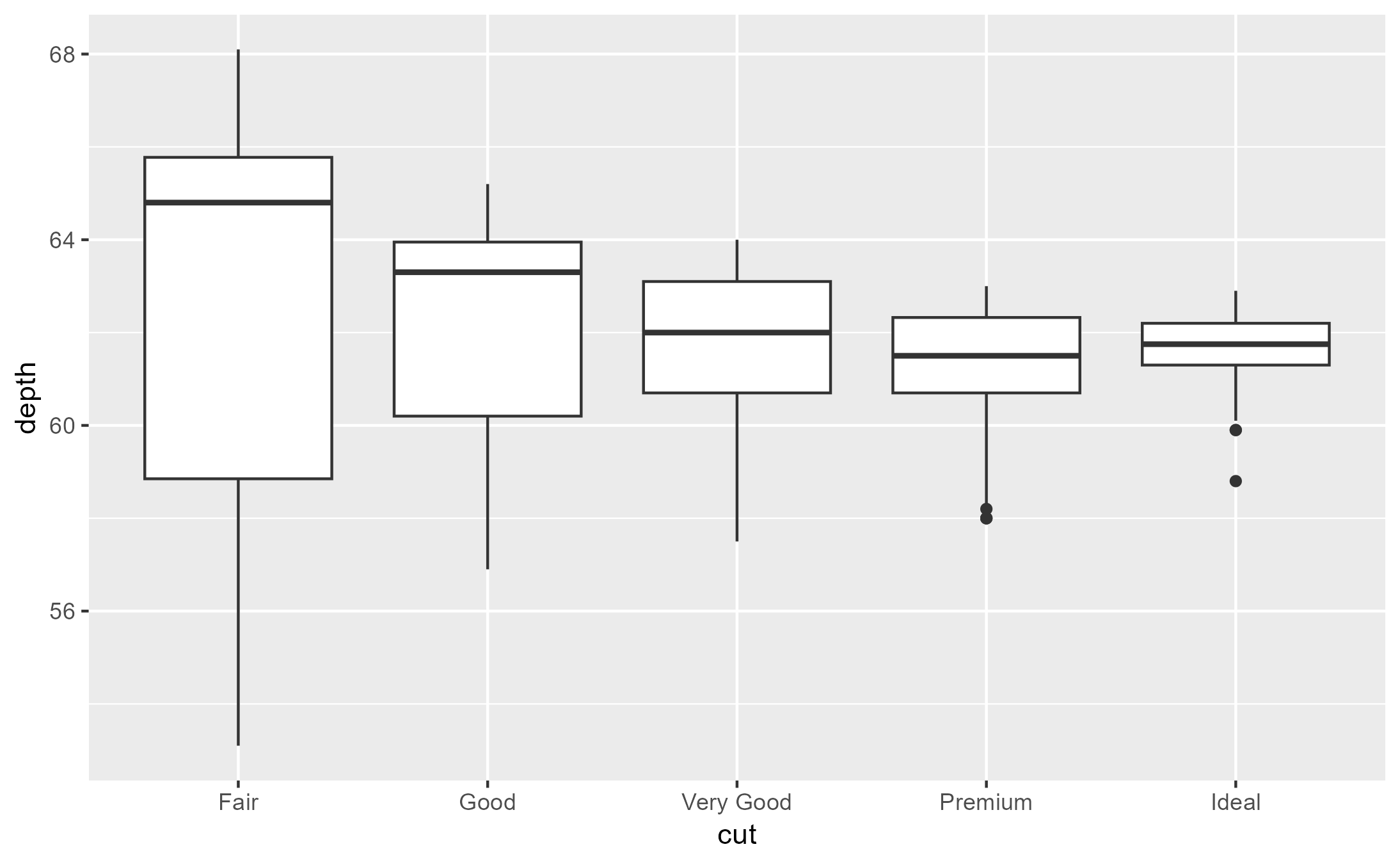first_tracedump <- last_ggtrace() # Short-form functional with_ggtrace() method: second_tracedump <- with_ggtrace( x = boxplot_plot, method = StatBoxplot$compute_group,
trace_steps = -1, trace_exprs = quote(~step)
)

identical(first_tracedump, second_tracedump)
#>  TRUE

# An example with out = "gtable" (or "g")
grid_plot <- ggplot(mtcars, aes(mpg, hp)) +
geom_point() +
facet_grid(am ~ cyl)
grid_plot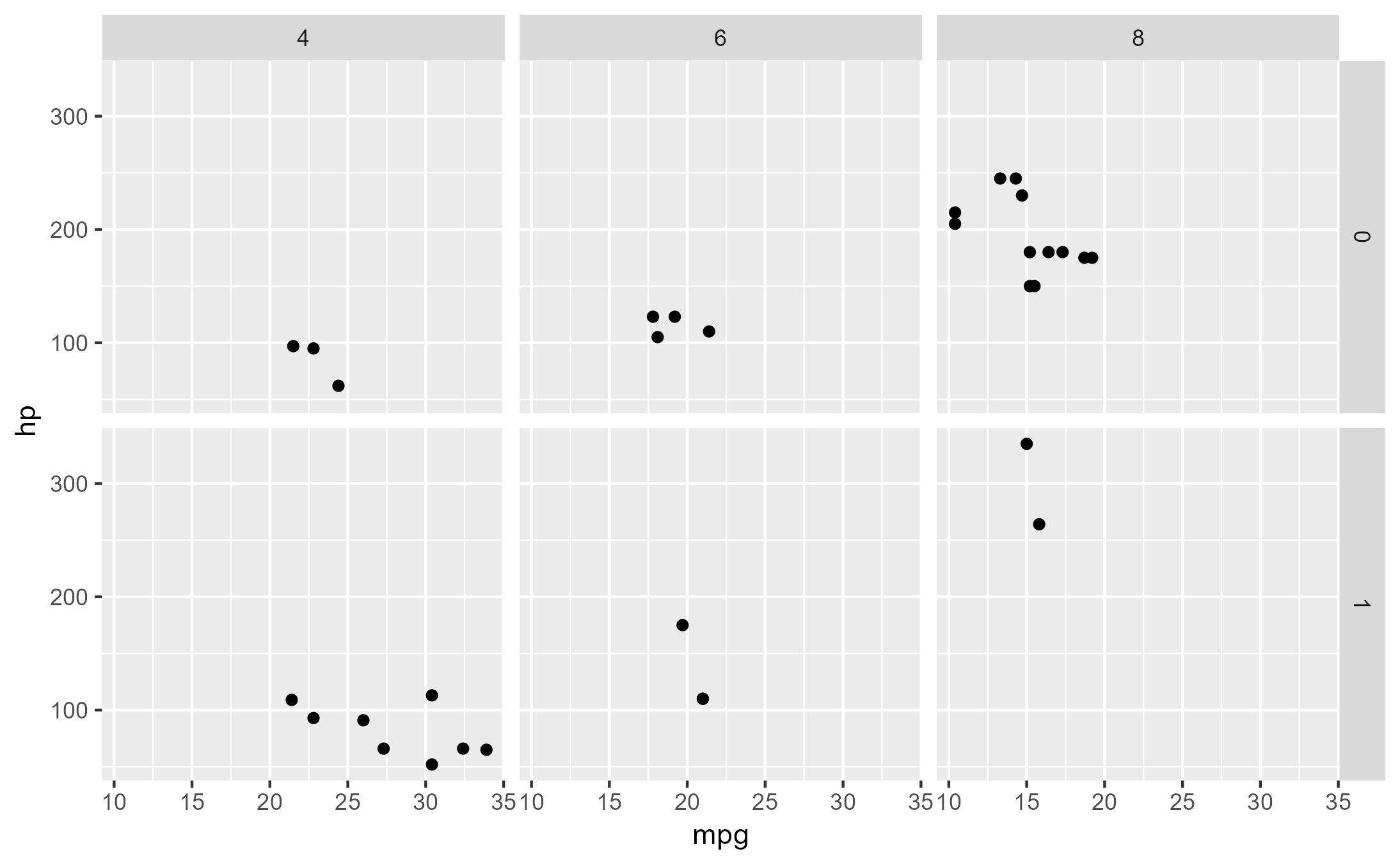outline <- grid::rectGrob(
x = 0.5, y = 0.5, width = 1, height = 1,
gp = grid::gpar(col = "red", lwd = 5, fill = NA)
)

with_ggtrace(
x = grid_plot,
method = Layout$render, trace_steps = 5, trace_exprs = rlang::expr({ panels[c(3, 5)] <- lapply(panels[c(3, 5)], function(panel) { gTree(children = gList(panel, !!outline)) }) }), out = "gtable" # or "g" )# With once = FALSE for persistent tracing (still cleaned up after) lm_plot <- ggplot(mpg, aes(displ, hwy, color = drv)) + geom_point() + geom_smooth(method = "lm") lm_plot #> geom_smooth() using formula = 'y ~ x'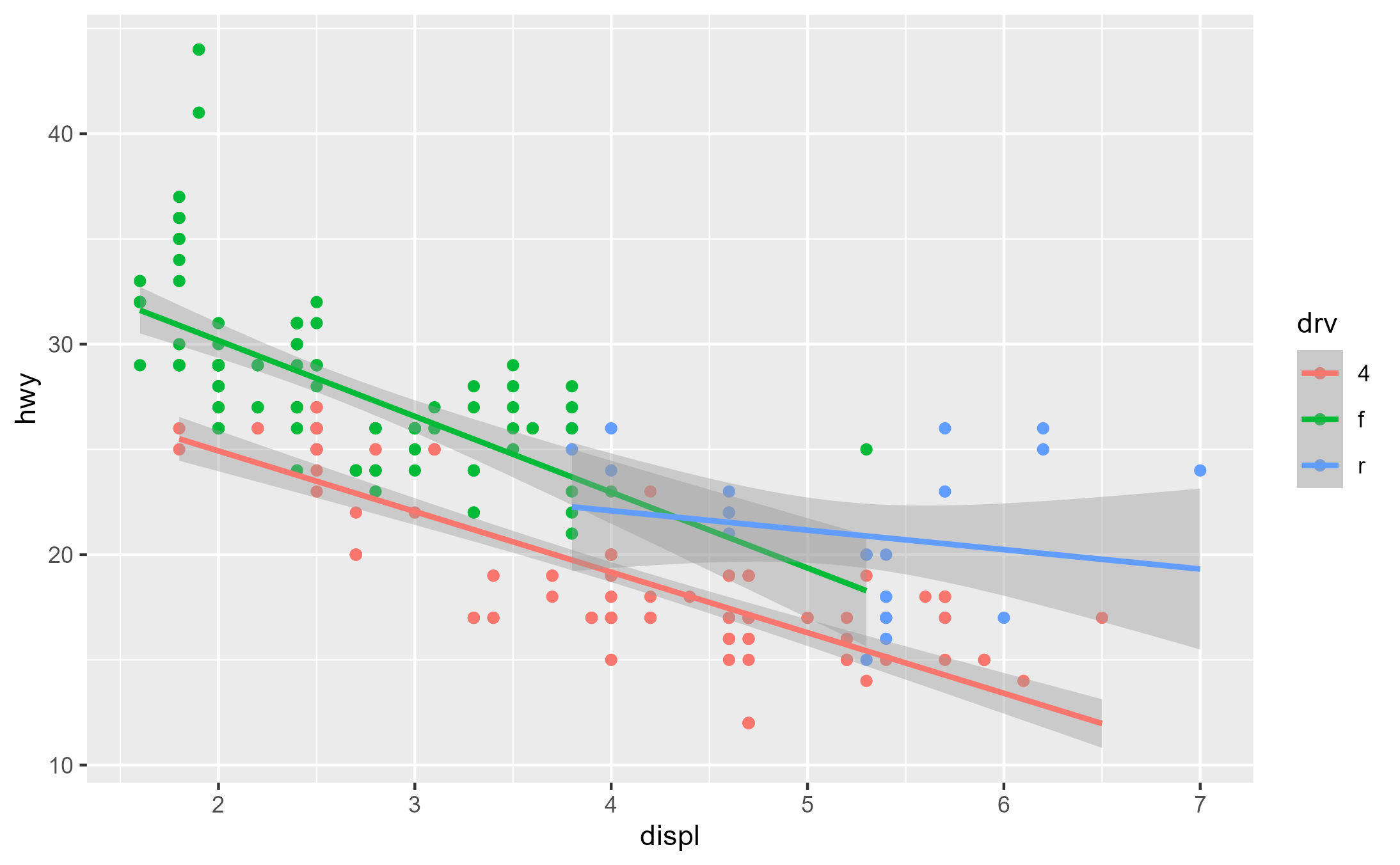with_ggtrace( x = lm_plot, method = StatSmooth$compute_group,
trace_steps = c(1, 11),
trace_exprs = list(
group = quote(data$group), coef = quote(model$coef)
)
)
#> $group #>  1 #> #>$coef
#> (Intercept)           x
#>   30.683113   -2.878486
#>

with_ggtrace(
x = lm_plot,
method = StatSmooth$compute_group, trace_steps = 1, trace_exprs = quote(method <- c("loess", "lm", "loess")[data$group]),
out = "g" # or "gtable"
)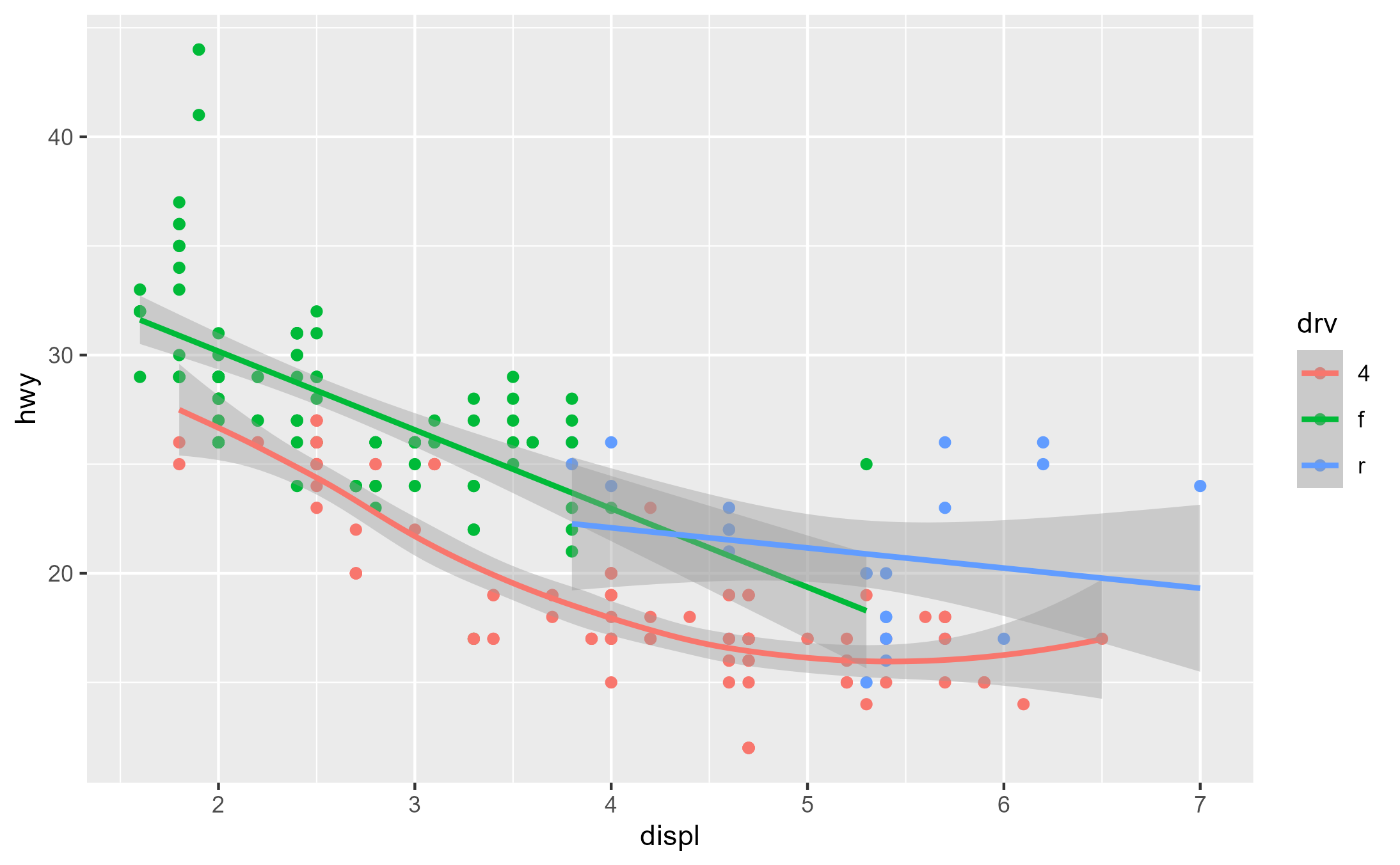# with_ggtrace() is useful for making calculations that are parasitic
# on a plot's execution environment.

library(grid)

square_plot <- ggplot(mtcars, aes(mpg, hp, color = factor(cyl))) +
geom_point() +
theme(aspect.ratio = 1)
square_plot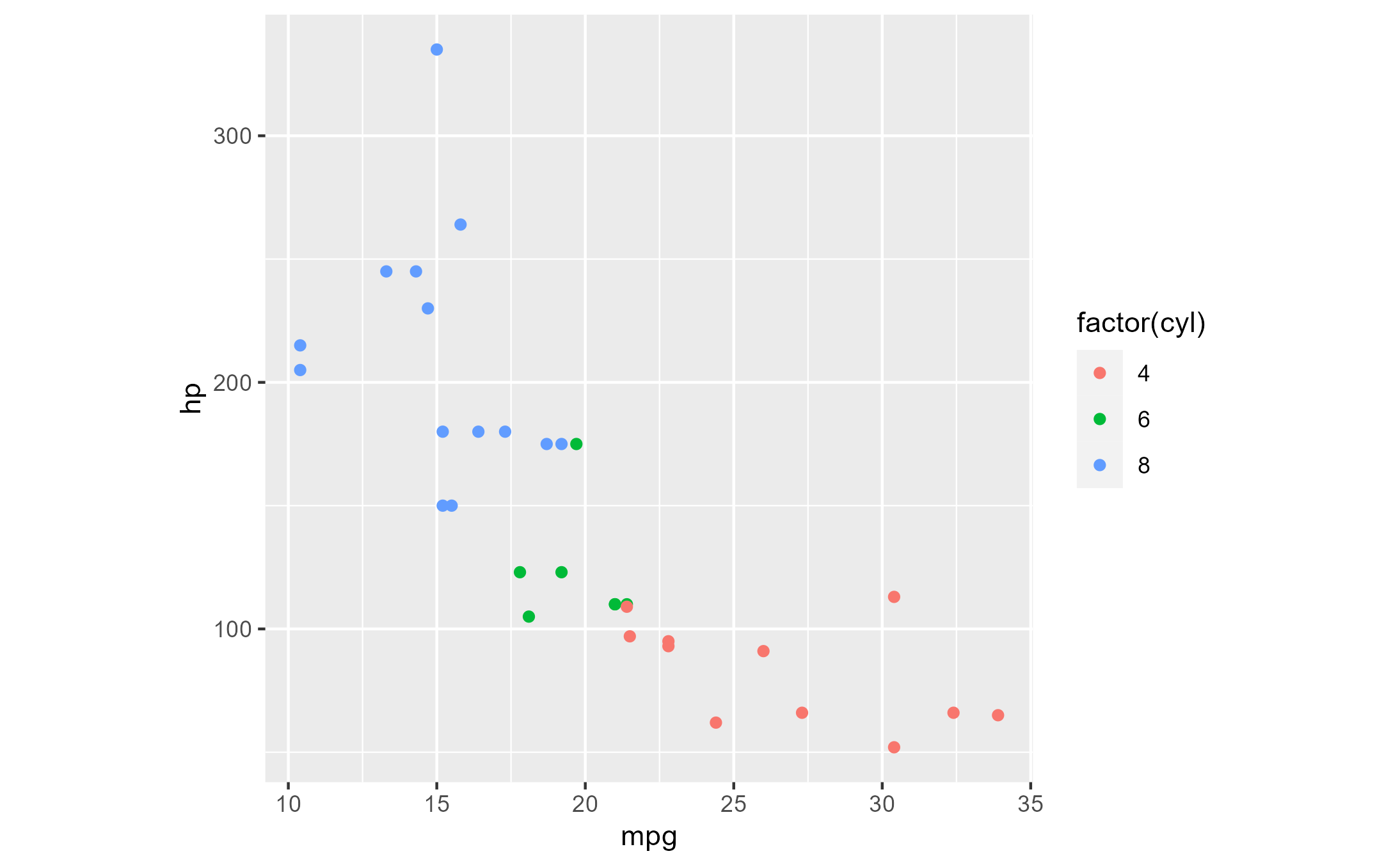rotation_vp <- viewport(width = .7, height = .7, angle = 45)

parasitic_evaluations <- with_ggtrace(
x = square_plot,
method = ggplot2:::ggplot_gtable.ggplot_built,
trace_steps = c(9, 13, -1),
trace_exprs = rlang::exprs(
plot_tbl = .plot_table <- editGrob(plot_table, vp = !!rotation_vp),
legend   = .legend <- editGrob(legend_box, vp = viewport(x = 0.15, y = 0.8)),
modified = gTree(children = gList(.plot_table, .legend))
)
)

grid.newpage()
grid.draw(parasitic_evaluations\$modified)
#> Warning: cannot clip to rotated viewport